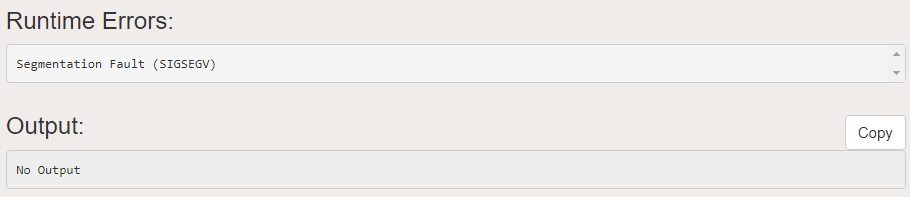# How to return a Pointer from a Function in C

• Difficulty Level : Basic
• Last Updated : 19 Aug, 2020

Pointers in C programming language is a variable which is used to store the memory address of another variable. We can pass pointers to the function as well as return pointer from a function. But it is not recommended to return the address of a local variable outside the function as it goes out of scope after function returns.

Program 1:

We provide nothing but the best curated videos and practice problems for our students. Check out the C Foundation Course and master the C language from basic to advanced level. Wait no more, start learning today!

The below program will give segmentation fault since ‘A’ was local to the function:

## C

 `// C program to illustrate the concept of ` `// returning pointer from a function ` `#include ` ` `  `// Function returning pointer ` `int``* fun() ` `{ ` `    ``int` `A = 10; ` `    ``return` `(&A); ` `} ` ` `  `// Driver Code ` `int` `main() ` `{ ` `    ``// Declare a pointer ` `    ``int``* p; ` ` `  `    ``// Function call ` `    ``p = fun(); ` ` `  `    ``printf``(``"%p\n"``, p); ` `    ``printf``(``"%d\n"``, *p); ` `    ``return` `0; ` `} `

Output:
Below is the output of the above program:Explanation:

The main reason behind this scenario is that compiler always make a stack for a function call. As soon as the function exits the function stack also gets removed which causes the local variables of functions goes out of scope.

Static Variables have a property of preserving their value even after they are out of their scope. So to execute the concept of returning a pointer from function in C you must define the local variable as a static variable.

Program 2:

## C

 `// C program to illustrate the concept of ` `// returning pointer from a function ` `#include ` ` `  `// Function that returns pointer ` `int``* fun() ` `{ ` `    ``// Declare a static integer ` `    ``static` `int` `A = 10; ` `    ``return` `(&A); ` `} ` ` `  `// Driver Code ` `int` `main() ` `{ ` `    ``// Declare a pointer ` `    ``int``* p; ` ` `  `    ``// Function call ` `    ``p = fun(); ` ` `  `    ``// Print Address ` `    ``printf``(``"%p\n"``, p); ` ` `  `    ``// Print value at the above address ` `    ``printf``(``"%d\n"``, *p); ` `    ``return` `0; ` `} `

Output:

```0x601038
10
```

My Personal Notes arrow_drop_up
Recommended Articles
Page :